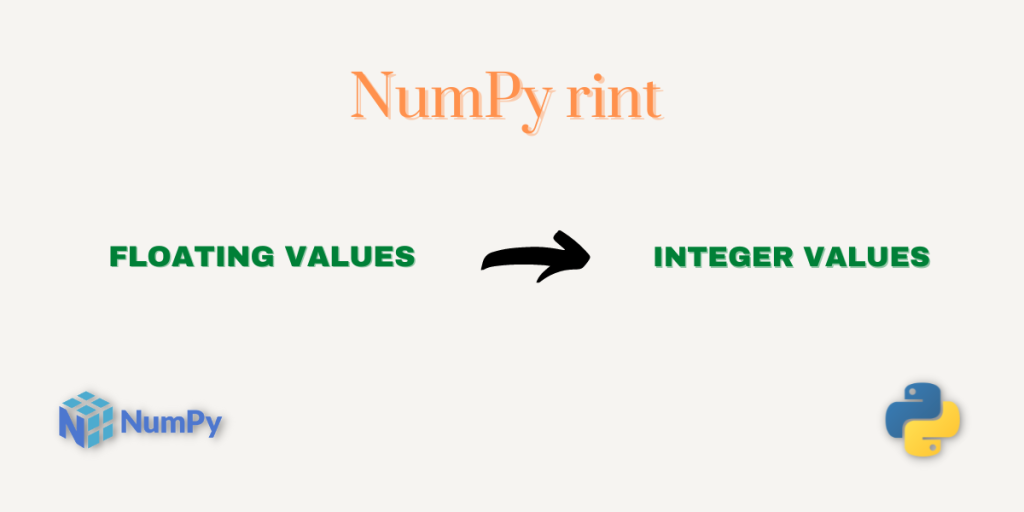# numpy.rint() – Round the NumPy Array Elements to the Nearest IntegerHello everyone! In this tutorial, we will be studying the NumPy `rint` function. This function is simple to understand and easy to use. Let’s get started.

## What is numpy.rint()?

NumPy `rint` is another mathematical function provided by the NumPy Library which rounds the input number to the nearest integer.

Let’s look at the syntax of this function.

## Syntax of numpy.rint()

```numpy.rint(input)
```

Here, the input can be a single number or a NumPy array of numbers. It’s important to note that the array returned by the `numpy.rint()` function has same type and shape as the input array.

## Working with the NumPy rint

We are done with the syntax part :). Let’s now write some code to understand this function better.

### numpy.rint() for single number as input

```import numpy as np

# Rounding the input values to the nearest integer
print("Rounded Value of 0.5 is:",np.rint(0.5))

print("Rounded Value of 1.5 is:",np.rint(1.5))

print("Rounded Value of 1.74 is:",np.rint(1.74))

print("Rounded Value of 5.56 is:",np.rint(5.56))

print("Rounded Value of 5.243 is:",np.rint(5.243))

print("Rounded Value of 10.111 is:",np.rint(10.111))

print("Rounded Value of 10.179 is:",np.rint(10.179))
```

Output

```Rounded Value of 0.5 is: 0.0
Rounded Value of 1.5 is: 2.0
Rounded Value of 1.74 is: 2.0
Rounded Value of 5.56 is: 6.0
Rounded Value of 5.243 is: 5.0
Rounded Value of 10.111 is: 10.0
Rounded Value of 10.179 is: 10.0
```

There is one interesting thing to observe is that the output of the `numpy.rint()` function is always an integer value. Let’s now try using the function with NumPy Array.

### NumPy rint for a NumPy array of Numbers

```import numpy as np

a = np.array((0.52 , 2.55 , 4.51 , 7.56 , 19.32))

print("Input Array:\n",a)
print("Rounded Values:\n",np.rint(a))
```

Output

```Input Array:
[ 0.52  2.55  4.51  7.56 19.32]
Rounded Values:
[ 1.  3.  5.  8. 19.]
```

The output array is pretty much obvious where the elements of the input array are rounded to the nearest integer values.

That was all about the NumPy rint function. Do check out amazing articles on other topics of python here.

## Reference

NumPy Documentation – NumPy rint